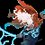# Finding The Inverse Tangent Based On The Definition Of Tangent Derived From Euler's Formula

In the section https://brilliant.org/wiki/eulers-formula/#trigonometric-applications, tan(x) is defined as (e^(i * x) - e^(-i * x)) / i * (e^(i * x) + e^(-i * x)). I have been trying to find the inverse tan(x) from this definition, but have been unable to do so. I first wrote the problem as y = (e^(i * x) - e^(-i * x)) / i * (e^(i * x) + e^(-i * x)), y being the tan(x), and then substituted all of the x for y and y for x. I now had the problem x = (e^(i * y) - e^(-i * y)) / i * (e^(i * y) + e^(-i * y)). I multiplied both sides by i and got x * i = (e^(i * y) - e^(-i * y)) / (e^(i * y) + e^(-i * y)), and then, knowing that a^(-b) = 1 / a^(b), rewrote the problem as x * i = (e^(i * y) - (1 / e^(i * y))) / (e^(i * y) + (1 / e^(i * y))), and combined terms from there to get x * i = ((e^(2 * i * y) - 1) / (e^(i * y))) / ((e^(2* i * y) + 1) / (e^(i * y))). I then divided the numerator's fraction by the denominator's fraction to get x * i = (e^(2 * i * y) - 1) / (e^(2 * i * y) + 1). From here, I do not know how to proceed. My goal is to isolate the y. Any suggestions on how I might do this would be greatly appreciated.Note by Michael Vonica
3 years, 11 months ago

This discussion board is a place to discuss our Daily Challenges and the math and science related to those challenges. Explanations are more than just a solution — they should explain the steps and thinking strategies that you used to obtain the solution. Comments should further the discussion of math and science.

When posting on Brilliant:

• Use the emojis to react to an explanation, whether you're congratulating a job well done , or just really confused .
• Ask specific questions about the challenge or the steps in somebody's explanation. Well-posed questions can add a lot to the discussion, but posting "I don't understand!" doesn't help anyone.
• Try to contribute something new to the discussion, whether it is an extension, generalization or other idea related to the challenge.
• Stay on topic — we're all here to learn more about math and science, not to hear about your favorite get-rich-quick scheme or current world events.

MarkdownAppears as
*italics* or _italics_ italics
**bold** or __bold__ bold
- bulleted- list
• bulleted
• list
1. numbered2. list
1. numbered
2. list
Note: you must add a full line of space before and after lists for them to show up correctly
paragraph 1paragraph 2

paragraph 1

paragraph 2

[example link](https://brilliant.org)example link
> This is a quote
This is a quote
    # I indented these lines
# 4 spaces, and now they show
# up as a code block.

print "hello world"
# I indented these lines
# 4 spaces, and now they show
# up as a code block.

print "hello world"
MathAppears as
Remember to wrap math in $$ ... $$ or $ ... $ to ensure proper formatting.
2 \times 3 $2 \times 3$
2^{34} $2^{34}$
a_{i-1} $a_{i-1}$
\frac{2}{3} $\frac{2}{3}$
\sqrt{2} $\sqrt{2}$
\sum_{i=1}^3 $\sum_{i=1}^3$
\sin \theta $\sin \theta$
\boxed{123} $\boxed{123}$

## Comments

Sort by:

Top Newest

Let y = e^(ix) first, then rearrange the equation to get a quadratic expression in terms of y.

- 3 years, 11 months ago

Log in to reply

Thanks Pi Han Goh. That lead me to my answer.

- 3 years, 11 months ago

Log in to reply

×

Problem Loading...

Note Loading...

Set Loading...